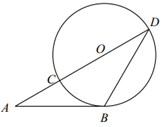$\text{A.}$ 3 $\text{B.}$ 4 $\text{C.}$ $3 \sqrt{3}$ $\text{D.}$ $4 \sqrt{2}$